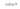# Generating Random Number between two numbers in JavaScriptIn this tutorial, we are going to learn about how to generate a random number between two numbers inclusively in JavaScript.

We can use `Math.floor` and `Math.random()` method to generate a random number between two numbers where both minimum and the maximum value is included in the output.

This below example shows you a function that is used to generate a random number based on the arguments we passed to it.

``````function randomNumber(min, max) { // min and max included
return Math.floor(Math.random() * (max - min+1)+min);
}

console.log(randomNumber(20, 25));``````

In the above code, we have passed `20`,`25` as an argument to `randomNumber` function so that we will get a random number between 20 to 25.

## Explanation

Let’s see how our `randomNumber` function works.

``Math.random()*(max-min+1)+min;``

First, we are multiplying `Math.random()` method with `(max-min+1)+min` values, so that we’ll get a random floating-point number between the `max` and `min` values.

Now we need to convert a floating-number to number or integer by passing it as an argument to the `Math.floor()` method.

The `Math.floor()` methods rounds the value to its nearest integer.

``````Math.floor(23.455); // 23

// Math.floor(Math.random()*(max-min+1)+min);

// Math.floor(Math.random()*(25-20+1)+20);

function randomNumber(min, max) {
return Math.floor(Math.random() * (max - min+1)+min);
}``````

## Top Udemy Courses##### JavaScript - The Complete Guide 2020 (Beginner + Advanced)
45,614 students enrolled
52 hours of video content
View Course##### React - The Complete Guide (incl Hooks, React Router, Redux)
284,472 students enrolled
40 hours of video content
View Course##### Vue JS 2 - The Complete Guide (incl. Vue Router & Vuex)
130,921 students enrolled
21 hours of video content
View Course